US6462885B2 - Zoom lens and photographing apparatus having the same - Google Patents

Zoom lens and photographing apparatus having the same Download PDF

Info

Publication number
US6462885B2
US6462885B2 US09/729,823 US72982300A US6462885B2 US 6462885 B2 US6462885 B2 US 6462885B2 US 72982300 A US72982300 A US 72982300A US 6462885 B2 US6462885 B2 US 6462885B2
Authority
US
United States
Prior art keywords
lens
lt
refractive power
subunit
represented
Prior art date
Legal status (The legal status is an assumption and is not a legal conclusion. Google has not performed a legal analysis and makes no representation as to the accuracy of the status listed.)
Active, expires
Application number
US09/729,823
Other versions
US20010022696A1 (en
Inventor
Akihiro Nishio
Current Assignee (The listed assignees may be inaccurate. Google has not performed a legal analysis and makes no representation or warranty as to the accuracy of the list.)
Canon Inc
Original Assignee
Canon Inc
Priority date (The priority date is an assumption and is not a legal conclusion. Google has not performed a legal analysis and makes no representation as to the accuracy of the date listed.)
Filing date
Publication date
Priority to JP11-351518 priority Critical
Priority to JP35151899A priority patent/JP4146977B2/en
Application filed by Canon Inc filed Critical Canon Inc
Assigned to CANON KABUSHIKI KAISHA reassignment CANON KABUSHIKI KAISHA ASSIGNMENT OF ASSIGNORS INTEREST (SEE DOCUMENT FOR DETAILS). Assignors: NISHIO, AKIHIRO
Publication of US20010022696A1 publication Critical patent/US20010022696A1/en
Application granted granted Critical
Publication of US6462885B2 publication Critical patent/US6462885B2/en
Application status is Active legal-status Critical
Adjusted expiration legal-status Critical

• 230000004075 alteration Effects 0 description 55
• 239000002131 composite material Substances 0 claims description 10
• 230000000875 corresponding Effects 0 description 20
• 238000006073 displacement Methods 0 description 5
• 230000000694 effects Effects 0 description 3
• 230000001747 exhibited Effects 0 description 1
• 239000010408 films Substances 0 description 2
• 230000014509 gene expression Effects 0 description 1
• 239000011521 glass Substances 0 description 2
• 238000003384 imaging method Methods 0 description 4
• 230000001965 increased Effects 0 description 3
• 239000000463 materials Substances 0 description 1
• 238000009740 moulding (composite fabrication) Methods 0 description 3
• 239000010950 nickel Substances 0 description 1
• 230000003287 optical Effects 0 abstract claims description 41
• 230000002093 peripheral Effects 0 description 1
• 230000002265 prevention Effects 0 description 7
• 230000003449 preventive Effects 0 description 1
• 230000011514 reflex Effects 0 description 3
• 230000001629 suppression Effects 0 description 2

Images

•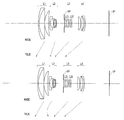•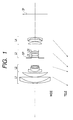••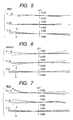•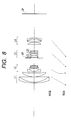•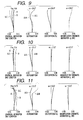•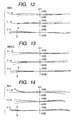•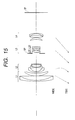•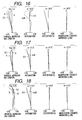•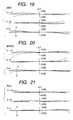•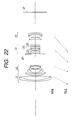•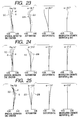•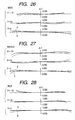•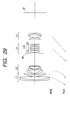•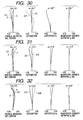•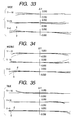•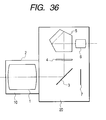Classifications

• GPHYSICS
• G02OPTICS
• G02BOPTICAL ELEMENTS, SYSTEMS, OR APPARATUS
• G02B27/00Other optical systems; Other optical apparatus
• G02B27/64Imaging systems using optical elements for stabilisation of the lateral and angular position of the image
• G02B27/646Imaging systems using optical elements for stabilisation of the lateral and angular position of the image compensating for small deviations, e.g. due to vibration or shake
• GPHYSICS
• G02OPTICS
• G02BOPTICAL ELEMENTS, SYSTEMS, OR APPARATUS
• G02B15/00Optical objectives with means for varying the magnification
• G02B15/14Optical objectives with means for varying the magnification by axial movement of one or more lenses or groups of lenses relative to the image plane for continuously varying the equivalent focal length of the objective
• G02B15/16Optical objectives with means for varying the magnification by axial movement of one or more lenses or groups of lenses relative to the image plane for continuously varying the equivalent focal length of the objective with interdependent non-linearly related movements between one lens or lens group, and another lens or lens group
• G02B15/163Optical objectives with means for varying the magnification by axial movement of one or more lenses or groups of lenses relative to the image plane for continuously varying the equivalent focal length of the objective with interdependent non-linearly related movements between one lens or lens group, and another lens or lens group having a first movable lens or lens group and a second movable lens or lens group, both in front of a fixed lens or lens group
• G02B15/167Optical objectives with means for varying the magnification by axial movement of one or more lenses or groups of lenses relative to the image plane for continuously varying the equivalent focal length of the objective with interdependent non-linearly related movements between one lens or lens group, and another lens or lens group having a first movable lens or lens group and a second movable lens or lens group, both in front of a fixed lens or lens group having an additional fixed front lens or group of lenses
• G02B15/173Optical objectives with means for varying the magnification by axial movement of one or more lenses or groups of lenses relative to the image plane for continuously varying the equivalent focal length of the objective with interdependent non-linearly related movements between one lens or lens group, and another lens or lens group having a first movable lens or lens group and a second movable lens or lens group, both in front of a fixed lens or lens group having an additional fixed front lens or group of lenses arranged +-+

Abstract

A zoom lens includes a first lens unit with a positive refractive power, a second lens unit with a negative refractive power, a third lens unit with a positive refractive power, and a fourth lens unit with a positive refractive power, which are arranged in this order from an object side. At least the first, third and fourth lens units are moved along an optical axis to vary spatial distances of the lens units to thereby change the magnification of the zoom lens. The third lens unit includes a first lens subunit with a positive refractive power, and a second lens subunit with a negative refractive power, and the second lens subunit is moved to have at least a perpendicular vector component with respect to the optical axis to thereby displace an image.

Description

BACKGROUND OF THE INVENTION

1. Field of the Invention

The present invention relates to a zoom lens having fluctuation prevention function and a photographing apparatus provided with the zoom lens. In particular, the present invention relates to a zoom lens having fluctuation prevention function and a photographing apparatus, which can be preferably applied to a still camera, a video camera, a digital camera, etc., and which is designed to allow a lens group, i.e., a part of the zoom lens, to be moved in a direction perpendicular to an optical axis so as to optically correct a blur of a photographed still image caused by the fluctuating motion (inclined motion) of the zoom lens due to hand fluctuation or the like, thereby obtaining a stabilized photographed image.

2. Related Background Art

Blur is caused on a photographed image due to hand fluctuation transmitted to a photographing system. Various attempts have been made to provide fluctuation preventive optical systems that can prevent the blur on the photographed image.

Recently, for a still camera, a video camera, a digital camera, etc., a zoom lens having fluctuation prevention function has been required to correct the image blur caused due to hand fluctuation or the like for the purpose of obtaining a higher quality image and enlarging applicable photographing conditions.

As a zoom lens having fluctuation prevention function, for example, Japanese Patent Application Laid-Open No. 9-230236 discloses a four-lens-group zoom lens in which positive, negative, positive and positive refractive power lens groups are arranged in this order from an object side, and in which the third lens group is divided into a positive refractive power front lens group and a positive refractive power rear lens group, and the rear lens group is moved perpendicularly to an optical axis to correct the image blur. Japanese Patent Application Laid-Open No. 10-232420 discloses a four-lens-group zoom lens, primarily applied to a video camera, in which positive, negative, positive and positive refractive power lens groups are arranged with the first and third lens groups to be fixed, and in which the third lens group is divided into a positive refractive power lens group and a negative refractive power lens group, and either one of these lens groups is moved in a direction perpendicular to an optical axis to correct image blur.

Japanese Patent Application Laid-Open No. 7-128619 discloses a magnification changeable optical system having four lens groups, which is provided, sequentially from an object side, with a first group having a positive refractive power fixed during the change of magnification and the in-focusing condition, a second group with a negative refractive power, having a magnification changing function, an aperture diaphragm, a third group with a positive refractive power, and a fourth group with a positive refractive power, that has both correction a function for correcting an image plane that varies depending on the change of magnification, and an in-focusing function. In the system, the third group is constructed of two lens groups, i.e., a third-1 group with a negative refractive power, and a third-2 group with a positive refractive power, and the third-2 group is moved in a direction perpendicular to an optical axis to correct blur of the photographed image caused by fluctuation of the magnification changeable optical system.

Japanese Patent Application Laid-Open No. 7-199124 discloses a magnification changeable optical system constructed of four groups, i.e., positive, negative, positive and positive refractive power lens groups, in which the third lens group entirely is fluctuated perpendicularly to an optical axis for the purpose of fluctuation prevention.

In general, an optical system, which is designed to parallell offset a part of lens of a photographing system in a direction perpendicular to an optical axis to correct the image blur, can advantageously realize the correction of the image blur in a relatively simple fashion, but requires driving means for driving the lens to be moved. Further, the system provides the result in an increased amount of offset aberration occurring during fluctuation prevention.

For example, if a correction lens group for correcting the image blur is large in its number of constituting lenses and weight, a large torque is required when electrically driving the same. Further, if the correction lens group for correcting the image blur is set inappropriately, the correction optical system requires a large amount of its movement in order to obtain an image-blur correcting effect of a certain degree, making the entire optical system large in size.

SUMMARY OF THE INVENTION

An object of the present invention is to provide a zoom lens and a photographing apparatus using the zoom lens, in which a relatively small and light-weight lens group forming a part of the zoom lens is moved in a direction perpendicular to an optical axis to correct image blur due to fluctuation (inclination) of the zoom lens, and in which the lens arrangement for the zoom lens is optimized to realize a zoom lens that is compact in size, has a simple mechanism, is small in the load applied to driving means, and that can effectively correct certain image blurring with a small amount of displacement of the lens group.

To attain the above-noted object, the present invention provides, as one form thereof, a zoom lens which includes:

a first lens unit with a positive refractive power;

a second lens unit with a negative refractive power;

a third lens unit with a positive refractive power; and

a fourth lens unit with a positive refractive power,

in which at least the first, third and fourth lens units are moved along an optical axis to vary spatial distances of the lens units to thereby the change magnification,

and the third lens unit includes a first lens subunit with a positive refractive power, and a second lens subunit with a negative refractive power, and the second lens subunit is moved to have at least a perpendicular vector component with respect to the optical axis to thereby displace an image.

As a preferable form, the following conditions are satisfied:

0.35<F3/Fm<1

−0.9<F3/F32<−0.18

where focal lengths of the entire system at a wide angle end and a telephoto end are respectively represented by Fw and Ft, the focal length of the third lens unit is represented by F3, the focal length of the second lens subunit is represented by F32, and Fm=(Fw×Ft)½.

As a preferable form, the second lens subunit is moved to have a perpendicular vector component with respect to the optical axis to thereby correct image blur caused by fluctuation of the zoom lens.

As a preferable form, the following condition is satisfied:

−0.2<(Ra+Rb)/(Ra−Rb)<0.7

where the radius of curvature of a lens plane located closest to an image plane side within the first lens subunit is represented by Ra, and the radius of curvature of a lens plane located closest to the object side within the second lens subunit is represented by Rb.

As a preferable form, the following conditions are satisfied:

0.7<F1/Fm<2.8

0.15<|F2/Fm|<0.7

0.5<F4/Fm<2.0

where the focal length of an i-th lens unit of the lens units is represented by Fi.

As a preferable form, the second lens subunit includes one positive lens and one negative lens.

As a preferable form, the first lens subunit includes, from the object side, a composite lens with a positive refractive power, in which a meniscus-like negative lens having a concave surface on an image plane side is adhered to a positive lens, and a composite lens with a positive single lens or a positive refractive power, in which a positive lens is adhered to a negative lens.

As a preferable form, the second lens subunit includes a composite lens with a negative refractive power, in which a positive lens having a convex surface oriented to an image plane side is adhered to a negative lens having a concave surface oriented to the object side.

As a preferable form, the third lens unit further includes a third lens subunit having a negative or positive refractive power on an image plane side of the second lens subunit.

To attain the above-noted object, the present invention provides, as another form, a photographing apparatus which includes:

a first lens unit with a positive refractive power;

a second lens unit with a negative refractive power;

a third lens unit with a positive refractive power;

a fourth lens unit with a positive refractive power; and

a casing holding a zoom lens,

in which at least the first, third and fourth lens units are moved along an optical axis to vary spatial distances of the lens units to thereby change the magnification,

and the third lens unit includes a first lens subunit with a positive refractive power, and a second lens subunit with a negative refractive power, and the second lens subunit is moved to have at least a perpendicular vector component with respect to the optical axis to thereby displace an image.

As a preferable form, the following conditions are satisfied:

0.35<F3/Fm<1

−0.9<F3/F32<−0.18

where the focal lengths of the entire system at a wide angle end and a telephoto end are respectively represented by Fw and Ft, the focal length of the third lens unit is represented by F3, the focal length of the second lens subunit is represented by F32, and Fm=(Fw×Ft)½.

As a preferable form, the second lens subunit is moved to have a perpendicular vector component with respect to the optical axis to thereby correct image blur caused due to fluctuation of the zoom lens.

As a preferable form, the following condition is satisfied:

−0.2<(Ra+Rb)/(Ra−Rb)<0.7

where the radius of curvature of a lens plane located closest to an image plane side within the first lens subunit is represented by Ra, and the radius of curvature of a lens plane located closest to the object side within the second lens subunit is represented by Rb.

As a preferable form, the following conditions are satisfied:

0.7<F1/Fm<2.8

0.15<|F2/Fm|<0.7

0.5<F4/Fm<2.0

where the focal length of an i-th lens unit of the lens units is represented by Fi.

BRIEF DESCRIPTION OF THE DRAWINGS

FIG. 1 is a sectional view showing a lens of a wide angle end according to a numerical first embodiment of the present invention;

FIG. 2 is a view showing aberration at the wide angle end under a normal condition according to the numerical first embodiment of the present invention;

FIG. 3 is a view showing aberration at a middle under a normal condition according to the numerical first embodiment of the present invention;

FIG. 4 is a view showing aberration at a telephoto end under a normal condition according to the numerical first embodiment of the present invention;

FIG. 5 is a view showing aberration at the wide angle end with correction for image blur corresponding to an 0.3 degree field angle according to the numerical first embodiment of the present invention;

FIG. 6 is a view showing aberration at the middle with correction for image blur corresponding to a 0.3 degree field angle according to the numerical first embodiment of the present invention;

FIG. 7 is a view showing aberration at the telephoto end with correction for image blur corresponding to a 0.3 degree field angle according to the numerical first embodiment of the present invention;

FIG. 8 is a sectional view showing a lens of a wide angle end according to a numerical second embodiment of the present invention;

FIG. 9 is a view showing aberration at the wide angle end under a normal condition according to the numerical second embodiment of the present invention;

FIG. 10 is a view showing aberration at a middle under a normal condition according to the numerical second embodiment of the present invention;

FIG. 11 is a view showing aberration at a telephoto end under a normal condition according to the numerical second embodiment of the present invention;

FIG. 12 is a view showing aberration at the wide angle end with correction for an image blur corresponding to a 0.3 degree field angle according to the numerical second embodiment of the present invention;

FIG. 13 is a view showing aberration at the middle with correction for an image blur corresponding to a 0.3 degree field angle according to the numerical second embodiment of the present invention;

FIG. 14 is a view showing aberration at the telephoto end with correction for an image blur corresponding to a 0.3 degree field angle according to the numerical second embodiment of the present invention;

FIG. 15 is a sectional view showing a lens of a wide angle end according to a numerical third embodiment of the present invention;

FIG. 16 is a view showing aberration at the wide angle end under a normal condition according to the numerical third embodiment of the present invention;

FIG. 17 is a view showing aberration at the middle under a normal condition according to the numerical third embodiment of the present invention;

FIG. 18 is a view showing aberration at a telephoto end under a normal condition according to the numerical third embodiment of the present invention;

FIG. 19 is a view showing aberration at the wide angle end with correction for an image blur corresponding to a 0.3 degree field angle according to the numerical third embodiment of the present invention;

FIG. 20 is a view showing aberration at the middle with correction for an image blur corresponding to a 0.3 degree field angle according to the numerical third embodiment of the present invention;

FIG. 21 is a view showing aberration at the telephoto end with correction for an image blur corresponding to a 0.3 degree field angle according to the numerical third embodiment of the present invention;

FIG. 22 is a sectional view showing a lens of a wide angle end according to a numerical fourth embodiment of the present invention;

FIG. 23 is a view showing aberration at the wide angle end under a normal condition according to the numerical fourth embodiment of the present invention;

FIG. 24 is a view showing aberration at the middle under a normal condition according to the numerical fourth embodiment of the present invention;

FIG. 25 is a view showing aberration at a telephoto end under a normal condition according to the numerical fourth embodiment of the present invention;

FIG. 26 is a view showing aberration at the wide angle end with correction for an image blur corresponding to a 0.3 degree field angle according to the numerical fourth embodiment of the present invention;

FIG. 27 is a view showing aberration at the middle with correction for an image blur corresponding to a 0.3 degree field angle according to the numerical fourth embodiment of the present invention;

FIG. 28 is a view showing aberration at the telephoto end with correction for an image blur corresponding to a 0.3 degree field angle according to the numerical fourth embodiment of the present invention;

FIG. 29 is a sectional view showing a lens of a wide angle end according to a numerical fifth embodiment of the present invention;

FIG. 30 is a view showing aberration at the wide angle end under a normal condition according to the numerical fifth embodiment of the present invention;

FIG. 31 is a view showing aberration at a middle under a normal condition according to the numerical fifth embodiment of the present invention;

FIG. 32 is a view showing aberration at a telephoto end under a normal condition according to the numerical fifth embodiment of the present invention;

FIG. 33 is a view showing aberration at the wide angle end with correction for an image blur corresponding to a 0.3 degree field angle according to the numerical fifth embodiment of the present invention;

FIG. 34 is a view showing aberration at the middle with correction for an image blur corresponding to a 0.3 degree field angle according to the numerical fifth embodiment of the present invention;

FIG. 35 is a view showing aberration at the telephoto end with correction for an image blur corresponding to a 0.3 degree field angle according to the numerical fifth embodiment of the present invention; and

FIG. 36 is a schematic view showing major portions of a single reflex camera to which a zoom lens according to the present invention is applied.

DESCRIPTION OF THE PREFERRED EMBODIMENTS

FIGS. 1, 8, 15, 22 and 29 are sectional views respectively showing lenses at wide angle ends according to numerical first to fifth embodiments of the present invention. FIGS. 2 to 4 are views respectively showing aberration at the wide angle end, a middle, and a telephoto end under a normal condition according to the numerical first embodiment of the present invention. FIGS. 5 to 7 are views respectively showing aberration at the wide angle end, the middle, and the telephoto end in a fluctuation compensation state (correction for image blur corresponding to a 0.3 degree field angle) according to the numerical first embodiment of the present invention. FIGS. 9 to 11 are views respectively showing aberration at the wide angle end, the middle, and the telephoto end according to the numerical second embodiment of the present invention. FIGS. 12 to 14 are views respectively showing aberration at the wide angle end, the middle, and the telephoto end in a fluctuation compensation state (correction for image blur corresponding to a 0.3 degree field angle) according to the numerical second embodiment of the present invention. FIGS. 16 to 18 are views respectively showing aberration at the wide angle end, the middle, and the telephoto end under a normal condition according to the numerical third embodiment of the present invention. FIGS. 19 to 21 are views showing aberration at the wide angle end, the middle, and the telephoto end in a fluctuation compensation state (correction for image blur corresponding to a 0.3 degree field angle) according to the numerical third embodiment of the present invention. FIGS. 23 to 25 are views respectively showing aberration at the wide angle end, the middle and the telephoto end under a normal condition according to the numerical fourth embodiment of the present invention. FIGS. 26 to 28 are views respectively showing aberration at the wide angle end, the middle, and the telephoto end in a fluctuation compensation state (correction for image blur corresponding to a 0.3 degree field angle) according to the numerical fourth embodiment of the present invention. FIGS. 30 to 32 are views respectively showing aberration at the wide angle end, the middle, and the telephoto end under a normal condition according to the numerical fifth embodiment of the present invention. FIGS. 33 to 35 are views respectively showing aberration at the wide angle end, the middle, and the telephoto end in a fluctuation compensation state (correction for image blur corresponding to a 0.3 degree field angle) according to the numerical fifth embodiment of the present invention.

In the drawings, L1, L2, L3, and L4 respectively designate a first lens group with a positive refractive power, a second lens group with a negative refractive power, a third lens group with a positive refractive power, and a fourth lens group with a positive refractive power. Each arrow in the drawings shows a direction in which a respective lens group is moved when the magnification is changed from the wide angle side to the telephoto side. SP designates a diaphragm (stop) which is provided between the second and third lens groups. IP designates an image plane.

In each of FIGS. 1, 8, and 15, the third lens group includes a positive refractive power, third-first lens group L31, and a fluctuation-preventive, third second lens group L32 with a negative refractive power. In each of FIGS. 22 and 29, the third lens group includes a third-first lens group L31 with a positive refractive power, a fluctuation-preventive third-second lens group L32 with a negative refractive power, and a third-third lens group L33 with a negative refractive power.

Each of the zoom lenses according to the numerical embodiments of the present invention includes the first lens group with positive refractive power, the second lens group with the negative refractive power, the third lens group with the positive refractive power, and the fourth lens group with the positive refractive power arranged in this order from the object side, in which at least the first, third and fourth lens groups are moved along the optical axis to vary spatial distances therebetween for a magnification change, while the third-second lens group L32 with the negative refractive power in the third lens group is moved perpendicular to the optical axis to change the imaging position.

In the magnification change, the spatial distance between the first lens group and the second lens group is varied so that the second lens group mainly performs the magnification changing operation, and the third lens group is moved to mainly perform the correcting operation for the image plane varied in association with the magnification change, and concurrently the spatial distance between the third lens group and the fourth lens group is varied to correct a variation in decentered aberration in association with the magnification change. In this case, the second lens group may be fixed with respect to the optical axis to simplify the mechanism. By disposing the third-second lens group with the negative refractive power within the third lens group with the positive refractive power, the negative refractive function of the third second lens group cancels the various aberrations caused by a lens group having the positive refractive function, which is located as a lens system, other than the third-second lens group, within the third lens group. This also serves to provide a larger displacement of the image position with a smaller amount of movement.

In this case, the third lens group includes, from the object side, the third-first lens group L31 with the positive refractive power, and the third-second lens group with the negative refractive power, or the third-first lens group L31 with the positive refractive power, the third-second lens group with the negative refractive power, and the third-third lens group with the negative refractive power, and the third-second lens group is moved perpendicular to the optical axis to displace or vary the imaging position. With this arrangement, the converging effect of the third-first lens group renders a lens system of the third-second lens group small in size and allows a moving mechanism for the third-second lens group to be simplified.

Although a zoom lens aimed at the present invention can be realized by satisfying the above-noted conditions, it is preferable to satisfy at least one of the following conditions in order that the zoom lens exhibits excellent optical performance and the entire optical system is made smaller.

(A) If focal lengths of the entire system at the wide angle end and the telephoto end are respectively represented by Fw and Ft, a focal length of the third lens group is represented by F3, and a focal length of the third-second lens group is represented by F32, and if

Fm={square root over (Fw·Ft)},

then the following conditions are satisfied:

0.35<F3/Fm<1  (1)

−0.9<F3/F32<−0.18  (2)

In a case where the refractive power of the third lens group is weakened beyond the upper limit of the formula (1), the amount of the movement of the lens group for securing a certain focal length and magnification changing ratio becomes large, and thus, the entire length of the lens system becomes disadvantageously large.

On the other hand, if the lower limit is exceeded, the refractive power of the third lens group becomes excessively strong to generate the strong negative spherical aberration, making it difficult to appropriately correct the aberration over the entire range of the magnification by other lens groups.

The formula (2) relates to the refractive power of the third-second lens group performing the operation of displacing the imaging position within the third lens group, and should be satisfied in order to maintain high quality image while suppressing the movement of the third-second lens group.

In a case where the negative refractive power of the third-second lens group is weakened beyond the upper limit of the formula (2), the amount of the movement of the third-second lens group required for performing a certain image position displacement operation is large, and further the lens diameter of the third-second lens group is increased in order to obtain certain peripheral optical intensity. Thus, it is not preferable.

On the other hand, in a case where the lower limit is exceeded, the negative refractive power of the third-second lens group is large, and then the lens system, other than the third-second lens group, within the third lens group must have a larger positive refractive power, and therefore high-ordered spherical aberration or coma aberration is largely generated, making it difficult to correct the aberration when the image position is displaced.

In addition, it is more preferable to modify the conditions (1) and (2) as follows:

0.4<F3/Fm<0.8  (1a)

−0.8<F3/F32<−0.2  (2a)

(B) If a radius of curvature of a lens plane located the closest to the image plane side within the third-first lens group is represented by Ra, and a radius of curvature of a lens plane located the closest to the object side within the third-second lens group is represented by Rb,

then the following condition is satisfied:

−0.2<(Ra+Rb)/(Ra−Rb)<0.7  (3)

The formula (3) should be satisfied in order that the lens surface configurations are appropriately arranged within the third lens group for the purpose of obtaining a high quality image. Outside the numerical range defined by the formula (3), an appropriate canceling relationship is not established in spherical aberration and coma aberration by mutual lens surfaces when the image position is changed and not changed, and therefore is not preferable.

In addition, it is more preferable to modify the formula (3) as follows:

−0.15<(Ra+Rb)/(Ra−Rb)<0.6  (3a)

(C) If a focal length of an i-th lens group of the above-noted lens group is represented by Fi,

then the following conditions are satisfied:

0.7<F1/Fm<2.8  (4)

0.15<|F2/Fm|<0.7  (5)

0.5<F4/Fm<2.0  (6)

The formulae (4) to (6) are for the purpose of mainly realizing a compact optical system with high image quality.

If the lens system is outside the upper limit of the formula (4), the refractive power of the first lens group becomes excessively weak so that the lens diameter and the lens entire length become large, which is not preferable.

On the other hand, if the lens system is outside the lower limit, the refractive power of the first lens group becomes excessively strong, so that high-ordered spherical aberration is largely generated, making it difficult to correct the aberration.

If the lens system is outside the upper limit of the formula (5), the refractive power of the second lens group is weakened, so that the amount of the movement of each lens group is large in order to obtain certain magnification changing ratio, and consequently it is difficult to make the lens system compact in size.

Of the lens system is outside the lower limit, the negative refractive power function becomes large, so that the Petzval sum becomes a large negative value, and thus the image surface curvature is large, which is not preferable.

If the lens system is outside the upper limit of the formula (6), the refractive power of the fourth lens group, becomes too weak, so that the back focus is long, and thus the entire length of the lens is large, which is not preferable.

On the other hand, if the lens system is outside the lower limit, the back focus of the entire lens system becomes too short, so that in a case where the lens system is, for instance, applied to a single reflex lens, the interference with a quick return mirror occurs. Further, decentered, high-ordered aberration such as image plane curvature, is largely generated.

In addition, it is more preferable to modify the conditions (4), (5) and (6) as follows:

0.9<F1/Fm<2.3  (4a)

0.18<|F2/Fm|<0.6  (5a)

0.6<F4/Fm<1.8  (6a)

(D) The third-second lens group is preferably constituted by one positive lens and one negative lens. This is effective in suppressing aberration variation when the lens is moved to displace the image position.

(E) It is preferable that the focusing is carried out by moving the first lens group or the second lens group toward the object side. In particular, the system in which the second lens group is moved is preferable because the lens diameter of the first lens group is not increased. Both of the first and second lens groups may be moved to the object side to carry out the focusing.

(F) The first lens group is preferably constituted, from the object side, by a negative lens having a concave surface on the image plane side, which lens surface is stronger in refractive power than the that on the object side (hereafter, simply referred to as “the lens surface is stronger on the image plane side”, when applicable), a positive lens, and a positive lens having a convex surface on the object side, which lens surface is stronger in refractive power than that on the image plane side (hereafter, simply referred to as “the lens surface is stronger on the object side”, when applicable).

(G) The second lens group is preferably constituted, from the object side, by a negative lens having a concave surface which lens surface is stronger on the image plane side, a negative lens having concave surfaces on both sides, a positive lens having a convex surface which lens surface is stronger on the object side, and a negative lens having a concave surface which lens surface is stronger on the object side. Further, the negative lens located closest to the image plane side is preferably constructed as a composite lens of a negative lens and a positive lens to realize the higher image quality.

(H) The third-first lens group is preferably constituted, from the object side, by a composite lens in which a meniscus-like, negative lens having a concave surface stronger on the image plane side is adhered to a positive lens to constitute a lens group as being entirely positive, and a positive single lens or a composite lens in which a positive lens is adhered to a negative lens to constitute a lens group as being entirely positive.

(I) The third-second lens group is preferably constituted by a composite lens group in which a positive lens having a convex surface oriented to the image-plane side is adhered to a negative lens having a concave surface, which lens surface is stronger on the object side to constitute a lens group as being entirely negative.

(J) It is preferable to dispose, on the image plane side of the third-second lens group, a third-third lens group having a negative or positive refractive power, which is stationary during fluctuation prevention. With this arrangement, a further aberration correcting effect can be expected.

(K) The fourth lens group is preferably constituted, from the object side, by a positive lens having a convex surface stronger on the image plane side, a positive lens having convex surfaces on both sides, and a meniscus-like negative lens having a concave surface stronger on the object side.

(L) In order to improve the optical performance, a non-spherical surface, a grading optical element, and/or a gradient index optical element are preferably introduced into the lens system.

Next, specific numerical embodiments will be described.

In the numerical embodiments, Ri and Di respectively represent the thickness and the spatial distance of an i-th lens counted from the object side, and Ni and νi respectively represent the refractive power and the abbe number of the material of the i-th lens counted from the object side.

Non-spherical surface constants K, A, B, C, and D are defined by the following condition: $X=\text{ }\frac{{H}^{2}/R}{1+\sqrt{1-\left(1+K\right)\text{ }{\left(H/R\right)}^{2}}}+A·{H}^{2}+B·{H}^{4}+C·{H}^{6}+D·{H}^{8}+E·{H}^{10}$

where X denotes the amount of displacement in a direction from an optical axis to a lens apex, H denotes the distance from an optical axis, and R denotes the radius of curvature. Further, “e-X” means “x10−x”.

Relationships of numerical values of the numerical embodiments to aforementioned formulae are shown in Table 1.

Numerical Value Embodiment 1 f = 29.00˜101.37 Fno = 4.54˜5.75 2ω = 73.5°˜24.1° R1 = 86.687 D1 = 1.50 N1 = 1.846660 ν1 = 23.9 R2 = 42.162 D2 = 8.70 N2 = 1.603112 ν2 = 60.6 R3 = 467.815 D3 = 0.15 R4 = 36.727 D4 = 6.40 N3 = 1.733997 ν3 = 51.5 R5 = 125.386 D5 = variable R6 = 64.747 D6 = 1.20 N4 = 1.834807 ν4 = 42.7 R7 = 11.848 D7 = 5.34 R8 = −38.336 D8 = 1.00 N5 = 1.804000 ν5 = 46.6 R9 = 29.098 D9 = 0.20 R10 = 21.706 D10 = 3.10 N6 = 1.846660 ν6 = 23.9 R11 = −41.601 D11 = 0.60 R12 = −22.294 D12 = 1.00 N7 = 1.804000 ν7 = 46.6 R13 = −120.841 D13 = variable R14 = stop D14 = 0.15 R15 = 37.070 D15 = 0.90 N8 = 1.805181 ν8 = 25.4 R16 = 24.498 D16 = 3.00 N9 = 1.639300 ν9 = 44.9 R17 = −39.035 D17 = 0.20 R18 = 40.272 D18 = 3.30 N10 = 1.570989 ν10 = 50.8 R19 = −15.064 D19 = 0.90 N11 = 1.846660 ν11 = 23.9 R20 = −36.396 D20 = 1.73 R21 = −40.584 D21 = 2.00 N12 = 1.846660 ν12 = 23.9 R22 = −13.785 D22 = 0.90 N13 = 1.749497 ν13 = 35.3 R23 = 83.142 D23 = variable R24 = −132.327 D24 = 3.80 N14 = 1.487490 ν14 = 70.2 R25 = −22.027 D25 = 0.20 R26 = 110.113 D26 = 4.00 N15 = 1.487490 ν15 = 70.2 R27 = −41.738 D27 = 2.38 R28 = −19.597 D28 = 1.40 N16 = 1.846660 ν16 = 23.9 R29 = −33.679 focal distance variable range 29.00 54.74 101.37 D5 1.89 10.94 22.39 D13 12.99 6.28 1.45 D23 11.52 8.24 7.12

Numerical Value Embodiment 2 f = 29.00˜101.30 Fno = 4.39˜5.75 2ω = 73.5°˜24.1° R1 = 83.702 D1 = 1.50 N1 = 1.846660 ν1 = 23.9 R2 = 41.079 D2 = 8.40 N2 = 1.603112 ν2 = 60.6 R3 = 359.634 D3 = 0.15 R4 = 38.654 D4 = 6.40 N3 = 1.719995 ν3 = 50.2 R5 = 150.065 D5 = variable R6 = 64.696 D6 = 1.20 N4 = 1.834807 ν4 = 42.7 R7 = 12.027 D7 = 5.00 R8 = −37.123 D8 = 1.00 N5 = 1.804000 ν5 = 46.6 R9 = 28.835 D9 = 0.20 R10 = 21.702 D10 = 3.10 N6 = 1.846660 ν6 = 23.9 R11 = −39.022 D11 = 0.60 R12 = −22.580 D12 = 1.00 N7 = 1.804000 ν7 = 46.6 R13 = −120.841 D13 = variable R14 = stop D14 = 0.15 R15 = 37.553 D15 = 0.90 N8 = 1.805181 ν8 = 25.4 R16 = 25.970 D16 = 3.00 N9 = 1.639300 ν9 = 44.9 R17 = −45.144 D17 = 0.20 R18 = 41.663 D18 = 3.30 N10 = 1.570989 ν10 = 50.8 R19 = −14.860 D19 = 0.90 N11 = 1.846660 ν11 = 23.9 R20 = −38.349 D20 = 1.50 R21 = −42.901 D21 = 2.20 N12 = 1.846660 ν12 = 23.9 R22 = −13.652 D22 = 0.90 N13 = 1.749497 ν13 = 35.3 R23 = 91.409 D23 = variable R24 = −133.590 D24 = 3.80 N14 = 1.487490 ν14 = 70.2 R25 = −21.523 D25 = 0.20 R26 = 107.117 D26 = 3.80 N15 = 1.487490 ν15 = 70.2 R27 = −42.147 D27 = 2.60 R28 = −19.781 D28 = 1.40 N16 = 1.846660 ν16 = 23.9 R29 = −33.679 focal distance variable range 29.00 55.00 101.30 D5 2.00 10.87 22.39 D13 13.51 6.31 1.45 D23 11.52 8.30 7.12

Numerical Value Embodiment 3 f = 29.00˜101.50 Fno = 4.10˜5.75 2ω = 73.5°˜24.1° R1 = 141.446 D1 = 1.80 N1 = 1.846660 ν1 = 23.8 R2 = 65.548 D2 = 8.00 N2 = 1.603112 ν2 = 60.6 R3 = −1920.275 D3 = 0.15 R4 = 41.278 D4 = 5.00 N3 = 1.733997 ν3 = 51.5 R5 = 69.266 D5 = variable R6 = 26.753 D6 = 1.20 N4 = 1.834807 ν4 = 42.7 R7 = 12.779 D7 = 6.97 R8 = −111.739 D8 = 1.00 N5 = 1.804000 ν5 = 46.6 R9 = 27.679 D9 = 0.20 R10 = 20.183 D10 = 4.20 N6 = 1.846660 ν6 = 23.8 R11 = −229.709 D11 = 1.20 R12 = −34.939 D12 = 1.00 N7 = 1.804000 ν7 = 46.6 R13 = 343.160 D13 = variable R14 = stop D14 = 1.00 R15 = 382.311 D15 = 0.90 N8 = 1.805181 ν8 = 25.4 R16 = 13.917 D16 = 3.20 N9 = 1.639300 ν9 = 44.9 R17 = −70.454 D17 = 0.20 R18 = 26.018 D18 = 2.50 N10 = 1.720000 ν10 = 43.7 R19 = −51.100 D19 = 1.50 R20 = −45.633 D20 = 2.40 N11 = 1.846660 ν11 = 23.8 R21 = −15.099 D21 = 0.90 N12 = 1.749497 ν12 = 35.3 R22 = 225.399 D22 = variable R23 = −25.512 D23 = 3.00 N13 = 1.487490 ν13 = 70.2 R24 = −17.904 D24 = 0.20 R25 = 65.171 D25 = 4.00 N14 = 1.487490 ν14 = 70.2 R26 = −43.800 D26 = 3.14 R27 = −18.241 D27 = 1.40 N15 = 1.846660 ν15 = 23.8 R28 = −31.177 focal distance variable range 29.00 54.00 101.50 D5 1.80 15.36 29.97 D13 20.13 8.98 1.29 D22 14.01 10.76 9.85

Numerical Value Embodiment 4 f = 29.01˜101.35 Fno = 3.77˜5.80 2ω = 73.4°˜24.1° R1 = 126.261 D1 = 1.80 N1 = 1.846660 ν1 = 23.8 R2 = 66.940 D2 = 8.00 N2 = 1.603112 ν2 = 60.6 R3 = −282.096 D3 = 0.15 R4 = 37.686 D4 = 3.00 N3 = 1.670000 ν3 = 57.3 R5 = 46.991 D5 = variable R6 = 33.243 D6 = 1.20 N4 = 1.873996 ν4 = 35.3 R7 = 14.216 D7 = 6.40 R8 = −47.453 D8 = 1.00 N5 = 1.743198 ν5 = 49.3 R9 = 42.606 D9 = 0.20 R10 = 25.841 D10 = 4.20 N6 = 1.846660 ν6 = 23.8 R11 = −62.181 D11 = 1.02 R12 = −28.083 D12 = 1.00 N7 = 1.804000 ν7 = 46.6 R13 = −274.061 D13 = variable R14 = stop D14 = 1.00 R15 = 89.494 D15 = 0.90 N8 = 1.784723 ν8 = 25.7 R16 = 14.136 D16 = 4.00 N9 = 1.670000 ν9 = 57.3 R17 = −236.326 D17 = 0.20 R18 = 24.385 D18 = 3.30 N10 = 1.647689 ν10 = 33.8 R19 = −46.622 D19 = 1.00 R20 = −47.442 D20 = 2.40 N11 = 1.846660 ν11 = 23.8 R21 = −16.552 D21 = 0.90 N12 = 1.739997 ν12 = 31.7 R22 = −615.178 D22 = 1.80 R23 = −58.954 D23 = 1.80 N13 = 1.740999 ν13 = 52.6 *R24 = −211.512 D24 = variable R25 = −30.176 D25 = 3.00 N14 = 1.548141 ν14 = 45.8 R26 = −20.181 D26 = 0.20 R27 = 62.613 D27 = 5.00 N15 = 1.510091 ν15 = 63.6 R28 = −33.511 D28 = 2.07 R29 = −20.285 D29 = 1.40 N16 = 1.846660 ν16 = 23.8 R30 = −49.470 focal distance variable range 29.01 53.97 101.35 D5 1.80 14.59 29.63 D13 21.08 8.91 1.00 D24 11.12 9.28 9.18 aspherical coefficient 24 surfaces: k = −5.62727e+02 A = 0 B = 1.89300e−06 C = 3.98391e−08 D = −7.41272e−11 E = 0.00000e+00

Numerical Value Embodiment 5 f = 29.00˜101.50 Fno = 3.86˜5.75 2ω = 73.5°˜ 24.1° R1 = 233.840 D1 = 1.80 N1 = 1.846660 ν1 = 23.8 R2 = 98.856 D2 = 6.00 N2 = 1.658296 ν2 = 57.3 R3 = −305.171 D3 = 0.15 R4 = 51.919 D4 = 3.00 N3 = 1.603112 ν3 = 60.7 R5 = 80.974 D5 = variable R6 = 33.135 D6 = 1.20 N4 = 1.850259 ν4 = 32.3 R7 = 14.698 D7 = 6.73 R8 = −66.153 D8 = 1.00 N5 = 1.712995 ν5 = 53.9 R9 = 34.324 D9 = 0.20 R10 = 24.112 D10 = 4.20 N6 = 1.846660 ν6 = 23.8 R11 = −131.824 D11 = 1.35 R12 = −32.019 D12 = 1.00 N7 = 1.743198 ν7 = 49.3 R13 = −263.558 D13 = variable R14 = stop D14 = 1.00 R15 = 47.410 D15 = 0.90 N8 = 1.800999 ν8 = 35.0 R16 = 12.758 D16 = 4.30 N9 = 1.677900 ν9 = 55.3 R17 = 114.951 D17 = 0.20 R18 = 26.510 D18 = 3.00 N10 = 1.677900 ν10 = 55.3 R19 = −125.081 D19 = 1.30 R20 = −47.415 D20 = 3.00 N11 = 1.846660 ν11 = 23.8 R21 = −18.117 D21 = 0.90 N12 = 1.717362 ν12 = 29.5 R22 = −311.396 D22 = 1.80 R23 = 195.852 D23 = 1.80 N13 = 1.670000 ν13 = 57.3 *R24 = 7619.687 D24 = variable R25 = −30.835 D25 = 2.50 N14 = 1.568728 ν14 = 63.2 R26 = −21.996 D26 = 0.20 R27 = 72.912 D27 = 4.80 N15 = 1.518206 ν15 = 65.0 R28 = −28.835 D28 = 2.18 R29 = −18.744 D29 = 1.40 N16 = 1.850259 ν16 = 32.3 R30 = −51.138 focal distance variable range 29.00 54.00 101.50 D5 1.80 17.95 36.60 D13 23.15 10.02 1.35 D24 10.14 8.31 8.09 aspherical coefficient 24 surfaces: k = 1.81112e+04 A = 0 B = 1.77443e−06 C = −2.39986e−08 D = −5.47733e−11 E = 0.00000e+00

TABLE 1 Condition Numerical Value Embodiment expression 1 2 3 4 5 F3/Fm 0.594 0.650 0.445 0.700 0.586 F3/F32 −0.739 −0.736 −0.372 −0.380 −0.258 (Ra + Rb)/ −0.054 −0.056 0.057 −0.009 0.450 (Ra − Rb) F1/Fm 1.087 1.126 1.794 2.005 2.249 |F2/Fm| 0.226 0.234 0.342 0.370 0.413 F4/Fm 0.991 0.939 0.678 1.680 0.697

Next, an embodiment in which the zoom lens described above is applied to a photographing apparatus will be described with reference to FIG. 36.

FIG. 36 is a schematic diagram showing major portions of a single reflex camera. In FIG. 36, reference numeral 10 designates a photographing lens having the zoom lens 1 described above. The zoom lens 1 is held by a barrel 2, i.e. a holding member. Reference numeral 20 designates a camera main body which includes a quick return mirror 3 for reflecting rays of light from the photographing lens 10 upwardly, a focusing glass 4 disposed at a imaging forming position of the photographing lens 10, a pentadahaprism 5 for converting an inverted image formed on the focusing glass 4 into an erect image, and an eyepiece lens 6 or the like for observing the erect image. Reference numeral 7 designates a film plane. During photographing, the quick return mirror 3 is retracted from the optical path so that an image is formed on the film plane 7 by the photographing lens 10.

The zoom lens described above can be effectively applied to the photographing apparatus as described with reference to this embodiment.

According to the present invention, a relatively small and light-weight lens group forming a part of a zoom lens is moved in a direction perpendicular to an optical axis to correct image blur cause due to fluctuation (inclination) of a zoom lens. In this system, the lens arrangement for the zoom lens is optimized to realize the zoom lens which is compact in size, having a simple mechanism, having a small load when applied to driving means, and which can effectively correct a certain image blur with a small amount of displacement of the lens group.

Claims (16)

What is claimed is:
1. A zoom lens comprising:
a first lens unit with a positive refractive power;
a second lens unit with a negative refractive power;
a third lens unit with a positive refractive power; and
a fourth lens unit with a positive refractive power, which are arranged in this order from an object side,
wherein at least the first, third and fourth lens units are moved along an optical axis to vary spatial distances of the lens units to thereby change the magnification of the zoom lens,
wherein the third lens unit includes a first lens subunit with a positive refractive power, and a second lens subunit with a negative refractive power, and an image is displaced by moving said second lens subunit in such a way as to have a component perpendicular to an optical axis of said zoom lens.
2. A zoom lens according to claim 1, wherein said first lens subunit with the positive refractive power and said second lens subunit with the negative refractive power are arranged in this order from the object side.
3. A zoom lens according to claim 1, which satisfy the following conditions:
0.35<F3/Fm<1
−0.9<F3/F32<−0.18
where focal lengths of the entire system at a wide angle end and a telephoto end are respectively represented by Fw and Ft, the focal length of the third lens unit is represented by F3, the focal length of the second lens subunit is represented by F32, and Fm=(Fw×Ft)½.
4. A zoom lens according to claim 1, wherein the second lens subunit is moved to have a perpendicular vector component with respect to the optical axis to thereby correct image blur caused due to fluctuation of the zoom lens.
5. A zoom lens according to claim 1, which satisfy the following condition:
−0.2<(Ra+Rb)/(Ra−Rb)<0.7
where the radius of curvature of a lens plane located closest to an image plane side within the first lens subunit is represented by Ra, and the radius of curvature of a lens plane located closest to the object side within the second lens subunit is represented by Rb.
6. A zoom lens according to claim 1, which satisfy the following conditions:
0.7<F1/Fm<2.8
0.15<|F2/Fm|<0.7
0.5<F4/Fm<2.0
where the focal length of an i-th lens unit of the lens units is represented by Fi.
7. A zoom lens according to claim 1, wherein the second lens subunit includes a single positive lens and a single negative lens.
8. A zoom lens according to claim 1, wherein the first lens subunit includes, from the object side, a positive refractive power, composite lens in which a meniscus-like, negative lens having a concave surface on an image plane side is adhered to a positive lens, and a positive single lens or a positive refractive power, composite lens in which a positive lens is adhered to a negative lens.
9. A zoom lens according to claim 1, wherein the second lens subunit includes a negative refractive power, composite lens in which a positive lens having a convex surface oriented to an image plane side is adhered to a negative lens having a concave surface oriented to the object side.
10. A zoom lens according to claim 1, wherein the third lens unit further includes a third lens subunit having a negative or positive refractive power on an image plane side of the second lens subunit.
11. A photographing apparatus comprising:
the zoom lens according to claim 1; and
a casing holding the zoom lens.
12. A zoom lens according to claim 11, wherein said first lens subunit with the positive refractive power and said second lens subunit with the negative refractive power are arranged in this order from the object side.
13. A photographing apparatus according to claim 11, which satisfy the following conditions:
0.35<F3/Fm<1
−0.9<F3/F32<−0.18
where focal lengths of the entire system at a wide angle end and a telephoto end are respectively represented by Fw and Ft, the focal length of the third lens unit is represented by F3, the focal length of the second lens subunit is represented by F32, and Fm=(Fw×Ft)½.
14. A photographing apparatus according to claim 11, wherein the second lens subunit is moved to have a perpendicular vector component with respect to the optical axis to thereby correct image blur caused due to fluctuation of the zoom lens.
15. A photographing apparatus according to claim 11, which satisfy the following condition:
−0.2<(Ra+Rb)/(Ra−Rb)<0.7
where the radius of curvature of a lens plane located closest to an image plane side within the first lens subunit is represented by Ra, and the radius of curvature of a lens plane located closest to the object side within the second lens subunit is represented by Rb.
16. A photographing apparatus according to claim 11, which satisfy the following conditions:
0.7<F1/Fm<2.8
0.15<|F2/Fm|<0.7
0.5<F4/Fm<2.0
where the focal length of an i-th lens unit of the lens units is represented by Fi.
US09/729,823 1999-12-10 2000-12-06 Zoom lens and photographing apparatus having the same Active 2021-01-08 US6462885B2 (en)

Priority Applications (2)

Application Number Priority Date Filing Date Title
JP11-351518 1999-12-10
JP35151899A JP4146977B2 (en) 1999-12-10 1999-12-10 Zoom lens

Publications (2)

Publication Number Publication Date
US20010022696A1 US20010022696A1 (en) 2001-09-20
US6462885B2 true US6462885B2 (en) 2002-10-08

Family

Family Applications (1)

Application Number Title Priority Date Filing Date
US09/729,823 Active 2021-01-08 US6462885B2 (en) 1999-12-10 2000-12-06 Zoom lens and photographing apparatus having the same

Country Status (2)

US (1) US6462885B2 (en)
JP (1) JP4146977B2 (en)

Cited By (10)

* Cited by examiner, † Cited by third party
Publication number Priority date Publication date Assignee Title
US20050046962A1 (en) * 2003-08-11 2005-03-03 Norihiro Nanba Zoom lens system and imaging apparatus having the same
US6891398B2 (en) 2001-08-03 2005-05-10 Micron Technology, Inc. Skewed falling logic device for rapidly propagating a falling edge of an output signal
US20050157403A1 (en) * 2002-10-04 2005-07-21 Nikon Corporation Zoom lens system
US20060023317A1 (en) * 2004-07-30 2006-02-02 Canon Kabushiki Kaisha Zoom lens system and image pickup apparatus using the same
US20060072213A1 (en) * 2004-10-01 2006-04-06 Nikon Corporation Zoom lens system with vibration reduction
US20070091461A1 (en) * 2005-10-19 2007-04-26 Pentax Corporation Wide-angle zoom lens system
US20070133092A1 (en) * 2005-12-08 2007-06-14 Sony Corporation Lens device and imager apparatus
US20080019018A1 (en) * 2006-07-20 2008-01-24 Nikon Corporation Zoom lens system, imaging apparatus, method for vibration reduction, and method for varying focal length
US20140333821A1 (en) * 2013-05-10 2014-11-13 Canon Kabushiki Kaisha Zoom lens and image pickup apparatus including zoom lens
US9229203B2 (en) 2011-06-21 2016-01-05 Fujifilm Corporation Zoom lens and imaging apparatus

Families Citing this family (18)

* Cited by examiner, † Cited by third party
Publication number Priority date Publication date Assignee Title
JP2002333577A (en) * 2001-05-10 2002-11-22 Asahi Optical Co Ltd Vibration-proof observation optical system
JP3506691B2 (en) * 2002-02-20 2004-03-15 株式会社タムロン High-magnification zoom lens
JP2005266183A (en) * 2004-03-18 2005-09-29 Sigma Corp Telephotographic zoom lens
JP4806915B2 (en) * 2004-10-01 2011-11-02 株式会社ニコン Zoom lens with anti-vibration function
KR100671544B1 (en) 2005-06-10 2007-01-19 삼성전자주식회사 Zoom lens optical system
JP5125009B2 (en) * 2006-07-20 2013-01-23 株式会社ニコン Zoom lens, imaging device, zoom lens vibration isolation method, zoom lens zoom method
JP4949897B2 (en) * 2007-03-09 2012-06-13 富士フイルム株式会社 Zoom lens with image stabilization function and imaging device
EP2045637B1 (en) * 2007-10-02 2019-07-10 Nikon Corporation Zoom lens system
JP5126663B2 (en) * 2007-11-02 2013-01-23 株式会社ニコン Zoom lens and optical apparatus provided with the zoom lens
US8238038B2 (en) * 2008-08-08 2012-08-07 Tamron Co., Ltd. High variable power zoom lens
JP4807388B2 (en) * 2008-09-22 2011-11-02 株式会社ニコン Zoom lens with anti-vibration function
JP4905429B2 (en) * 2008-09-25 2012-03-28 株式会社ニコン Zoom lens
CN102292660B (en) * 2009-01-24 2014-07-16 株式会社尼康 Zoom lens, optical device provided with zoom lens and method for manufacturing zoom lens
JP5224468B2 (en) * 2009-05-12 2013-07-03 富士フイルム株式会社 Zoom lens and imaging device
JP5678424B2 (en) * 2009-11-26 2015-03-04 株式会社ニコン Variable magnification optical system, optical apparatus equipped with the variable magnification optical system, and method of manufacturing the variable magnification optical system
JP5426353B2 (en) * 2009-12-17 2014-02-26 株式会社シグマ Zoom lens with anti-vibration function
JP5722730B2 (en) * 2011-08-26 2015-05-27 株式会社タムロン Image stabilization zoom lens
EP3125011B1 (en) * 2014-03-27 2019-08-28 Nikon Corporation Variable power optical system, imaging device, and variable power optical system production method

Citations (19)

* Cited by examiner, † Cited by third party
Publication number Priority date Publication date Assignee Title
US5056900A (en) 1989-10-31 1991-10-15 Canon Kabushiki Kaisha Zoom lens
US5132848A (en) 1990-10-26 1992-07-21 Canon Kabushiki Kaisha Wideangle four group zoom lens
US5221994A (en) 1991-06-07 1993-06-22 Canon Kabushiki Kaisha Zoom lens of rear focus type
US5253113A (en) 1990-09-07 1993-10-12 Canon Kabushiki Kaisha Wide-angle zoom lens having five lens units
JPH07128619A (en) 1993-11-04 1995-05-19 Canon Inc Variable power optical system having vibrationproof function
JPH07199124A (en) 1993-12-28 1995-08-04 Nikon Corp Zoom lens with oscillation-proof function
US5523888A (en) 1993-09-03 1996-06-04 Canon Kabushiki Kaisha Zoom lens
US5574599A (en) 1992-11-18 1996-11-12 Canon Kabushiki Kaisha Zoom lens and zooming method
US5638216A (en) 1991-08-30 1997-06-10 Canon Kabushiki Kaisha Zoom lens
JPH09230236A (en) 1996-02-27 1997-09-05 Minolta Co Ltd Optical system provided with camera shake correcting function and zoom lens
US5691851A (en) 1993-07-14 1997-11-25 Canon Kabushiki Kaisha Zoom lens
JPH10232420A (en) 1997-02-20 1998-09-02 Matsushita Electric Ind Co Ltd Zoom lens
US5815320A (en) 1993-01-14 1998-09-29 Canon Kabushiki Kaisha Zoom lens
US5831772A (en) 1993-04-07 1998-11-03 Canon Kabushiki Kaisha Compact zoom lens
US6028716A (en) 1993-11-29 2000-02-22 Canon Kabushiki Kaisha Zoom lens
US6061180A (en) * 1996-10-29 2000-05-09 Canon Kabushiki Kaisha Zoom lens
US6061186A (en) 1997-01-21 2000-05-09 Canon Kabushiki Kaisha Zoom lens
US6115188A (en) 1997-10-16 2000-09-05 Canon Kabushiki Kaisha Optical system and optical apparatus having the same
US6141159A (en) 1997-07-11 2000-10-31 Canon Kabushiki Kaisha Viewfinder optical system

Patent Citations (21)

* Cited by examiner, † Cited by third party
Publication number Priority date Publication date Assignee Title
US5056900A (en) 1989-10-31 1991-10-15 Canon Kabushiki Kaisha Zoom lens
US5253113A (en) 1990-09-07 1993-10-12 Canon Kabushiki Kaisha Wide-angle zoom lens having five lens units
US5132848A (en) 1990-10-26 1992-07-21 Canon Kabushiki Kaisha Wideangle four group zoom lens
US5221994A (en) 1991-06-07 1993-06-22 Canon Kabushiki Kaisha Zoom lens of rear focus type
US5638216A (en) 1991-08-30 1997-06-10 Canon Kabushiki Kaisha Zoom lens
US5574599A (en) 1992-11-18 1996-11-12 Canon Kabushiki Kaisha Zoom lens and zooming method
US5815320A (en) 1993-01-14 1998-09-29 Canon Kabushiki Kaisha Zoom lens
US5831772A (en) 1993-04-07 1998-11-03 Canon Kabushiki Kaisha Compact zoom lens
US5691851A (en) 1993-07-14 1997-11-25 Canon Kabushiki Kaisha Zoom lens
US5523888A (en) 1993-09-03 1996-06-04 Canon Kabushiki Kaisha Zoom lens
JPH07128619A (en) 1993-11-04 1995-05-19 Canon Inc Variable power optical system having vibrationproof function
US6028716A (en) 1993-11-29 2000-02-22 Canon Kabushiki Kaisha Zoom lens
JPH07199124A (en) 1993-12-28 1995-08-04 Nikon Corp Zoom lens with oscillation-proof function
US5585966A (en) 1993-12-28 1996-12-17 Nikon Corporation Zoom lens with vibration reduction function
US6046852A (en) 1996-02-27 2000-04-04 Minolta Co., Ltd. Lens system with image blur correction
JPH09230236A (en) 1996-02-27 1997-09-05 Minolta Co Ltd Optical system provided with camera shake correcting function and zoom lens
US6061180A (en) * 1996-10-29 2000-05-09 Canon Kabushiki Kaisha Zoom lens
US6061186A (en) 1997-01-21 2000-05-09 Canon Kabushiki Kaisha Zoom lens
JPH10232420A (en) 1997-02-20 1998-09-02 Matsushita Electric Ind Co Ltd Zoom lens
US6141159A (en) 1997-07-11 2000-10-31 Canon Kabushiki Kaisha Viewfinder optical system
US6115188A (en) 1997-10-16 2000-09-05 Canon Kabushiki Kaisha Optical system and optical apparatus having the same

Cited By (17)

* Cited by examiner, † Cited by third party
Publication number Priority date Publication date Assignee Title
US6891398B2 (en) 2001-08-03 2005-05-10 Micron Technology, Inc. Skewed falling logic device for rapidly propagating a falling edge of an output signal
US7142370B2 (en) * 2002-10-04 2006-11-28 Nikon Corporation Zoom lens system
US20050157403A1 (en) * 2002-10-04 2005-07-21 Nikon Corporation Zoom lens system
US7023624B2 (en) * 2003-08-11 2006-04-04 Canon Kabushiki Kaisha Zoom lens system and imaging apparatus having the same
US20050046962A1 (en) * 2003-08-11 2005-03-03 Norihiro Nanba Zoom lens system and imaging apparatus having the same
US20060023317A1 (en) * 2004-07-30 2006-02-02 Canon Kabushiki Kaisha Zoom lens system and image pickup apparatus using the same
US7268954B2 (en) 2004-07-30 2007-09-11 Canon Kabushiki Kaisha Zoom lens system and image pickup apparatus using the same
US20060072213A1 (en) * 2004-10-01 2006-04-06 Nikon Corporation Zoom lens system with vibration reduction
US7253965B2 (en) * 2004-10-01 2007-08-07 Nikon Corporation Zoom lens system with vibration reduction
US7283311B2 (en) 2005-10-19 2007-10-16 Pentax Corporation Wide-angle zoom lens system
US20070091461A1 (en) * 2005-10-19 2007-04-26 Pentax Corporation Wide-angle zoom lens system
US20070133092A1 (en) * 2005-12-08 2007-06-14 Sony Corporation Lens device and imager apparatus
US20080019018A1 (en) * 2006-07-20 2008-01-24 Nikon Corporation Zoom lens system, imaging apparatus, method for vibration reduction, and method for varying focal length
US7492524B2 (en) 2006-07-20 2009-02-17 Nikon Corporation Zoom lens system, imaging apparatus, method for vibration reduction, and method for varying focal length
US9229203B2 (en) 2011-06-21 2016-01-05 Fujifilm Corporation Zoom lens and imaging apparatus
US20140333821A1 (en) * 2013-05-10 2014-11-13 Canon Kabushiki Kaisha Zoom lens and image pickup apparatus including zoom lens
US9664885B2 (en) * 2013-05-10 2017-05-30 Canon Kabushiki Kaisha Zoom lens and image pickup apparatus including zoom lens

Also Published As

Publication number Publication date
JP2001166208A (en) 2001-06-22
US20010022696A1 (en) 2001-09-20
JP4146977B2 (en) 2008-09-10

Similar Documents

Publication Publication Date Title
US6404561B1 (en) Taking lens apparatus
US6124984A (en) Variable magnification optical zoom lens system
US7023625B2 (en) Zoom lens and optical apparatus having the same
JP3359131B2 (en) The variable magnification optical system having a vibration reduction function
JP4626135B2 (en) Large-aperture-ratio inner-focusing telephoto zoom lens
JP5064837B2 (en) Zoom lens with anti-vibration function
JP4794912B2 (en) Zoom lens and imaging apparatus having the same
US20050270661A1 (en) Zoom lens system and image pickup apparatus having the same
US20020063961A1 (en) Variable magnification optical system having image stabilizing function
US4934796A (en) Zoom lens
US4927250A (en) Optical system for compensating chromatic abherration of image
US6456443B2 (en) Zoom lens system
JP5202003B2 (en) Zoom lens and imaging apparatus having the same
US4874231A (en) Zoom lens
US20010030809A1 (en) Zoom lens
US7990624B2 (en) Zoom lens and image pickup apparatus having the same
US5189557A (en) High variable magnification range zoom lens
US7184221B2 (en) Zoom lens system and camera incorporating the same
JP4978119B2 (en) High zoom ratio zoom lens
US6650475B1 (en) Variable power optical system and image pick-up apparatus having the same
JP3587272B2 (en) Zoom lens
US7315424B2 (en) Zoom lens and image pickup apparatus having the same
US7068428B2 (en) Zoom lens system and imaging apparatus having the same
US7126758B2 (en) Zoom lens system and image pickup apparatus having the same
JP5201809B2 (en) Zoom lens and imaging apparatus having the same

Legal Events

Date Code Title Description
AS Assignment

Owner name: CANON KABUSHIKI KAISHA, JAPAN

Free format text: ASSIGNMENT OF ASSIGNORS INTEREST;ASSIGNOR:NISHIO, AKIHIRO;REEL/FRAME:011639/0223

Effective date: 20010109

STCF Information on status: patent grant

Free format text: PATENTED CASE

CC Certificate of correction
CC Certificate of correction
CC Certificate of correction
FPAY Fee payment

Year of fee payment: 4

FPAY Fee payment

Year of fee payment: 8

FPAY Fee payment

Year of fee payment: 12